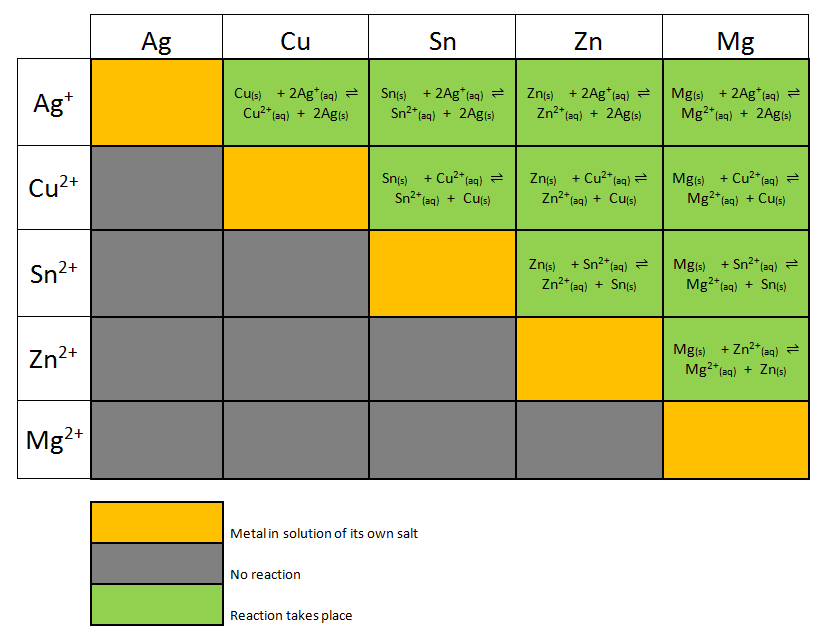# Electrochemistry

-Introduction

Step 1:

-Investigating redox reactions

-Practice with redox reactions

-Reduction tendencies of metal ions

Step 2:

-Electron transfer

-Electrochemical cell

-Practice with cells

-Powering a stopwatch

Step 3:

-Measuring potentials

-Calculating potentials

-Practice with potentials

-Applying potentials

Step 4:

-Non-standard conditions

-Practice with non-standard cells

Feedback Form:

Electrochemistry Tutorial: Galvanic Cells and the Nernst Equation >> Step 3: Applying standard cell potentials

 < Previous | Next >

# Electrochemistry: Galvanic Cells and the Nernst Equation

## Step 3: Applying standard cell potentials

Earlier in this activity, you ranked metal ions by their tendency to undergo reduction, where the tendency meant the ability of the metal ion to accept electrons from a metal. The ranking you found was:Look at the table of standard half-cell reduction potentials. What can you say about the relation between the table and the "reduction tendencies series" you derived above?

The greater the tendency of the ion to gain electrons and undergo reduction, the more positive the reduction potential of the ion. When we place a strip of metal in solution of another metal ion, the metal ion plates out on the strip if its reduction potential is greater (more positive) than the reduction potential of the ion of the other metal.

Recall the first reaction we investigated in this tutorial: copper ion has a greater (more positive) reduction potential than zinc, the metallic copper plates out on zinc. Mg2+ has the most negative potential and metallic magnesium will not plate out on any of the metals in the "reduction tendencies series".

The more positive the standard reduction potential, the more reactive the metal ion will be. The "reduction tendencies series" you created follows exactly the same order as the table of standard half-cell reduction potentials.

 < Previous | Next >